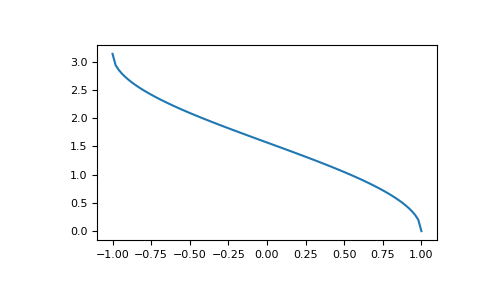# numpy.arccos¶

`numpy.``arccos`(x, /, out=None, *, where=True, casting='same_kind', order='K', dtype=None, subok=True[, signature, extobj]) = <ufunc 'arccos'>

Trigonometric inverse cosine, element-wise.

The inverse of `cos` so that, if `y = cos(x)`, then `x = arccos(y)`.

Parameters: x : array_like x-coordinate on the unit circle. For real arguments, the domain is [-1, 1]. out : ndarray, None, or tuple of ndarray and None, optional A location into which the result is stored. If provided, it must have a shape that the inputs broadcast to. If not provided or None, a freshly-allocated array is returned. A tuple (possible only as a keyword argument) must have length equal to the number of outputs. where : array_like, optional This condition is broadcast over the input. At locations where the condition is True, the out array will be set to the ufunc result. Elsewhere, the out array will retain its original value. Note that if an uninitialized out array is created via the default `out=None`, locations within it where the condition is False will remain uninitialized. **kwargs For other keyword-only arguments, see the ufunc docs. angle : ndarray The angle of the ray intersecting the unit circle at the given x-coordinate in radians [0, pi]. This is a scalar if x is a scalar.

`cos`, `arctan`, `arcsin`, `emath.arccos`

Notes

`arccos` is a multivalued function: for each x there are infinitely many numbers z such that cos(z) = x. The convention is to return the angle z whose real part lies in [0, pi].

For real-valued input data types, `arccos` always returns real output. For each value that cannot be expressed as a real number or infinity, it yields `nan` and sets the invalid floating point error flag.

For complex-valued input, `arccos` is a complex analytic function that has branch cuts [-inf, -1] and [1, inf] and is continuous from above on the former and from below on the latter.

The inverse `cos` is also known as acos or cos^-1.

References

M. Abramowitz and I.A. Stegun, “Handbook of Mathematical Functions”, 10th printing, 1964, pp. 79. http://www.math.sfu.ca/~cbm/aands/

Examples

We expect the arccos of 1 to be 0, and of -1 to be pi:

```>>> np.arccos([1, -1])
array([ 0.        ,  3.14159265])
```

Plot arccos:

```>>> import matplotlib.pyplot as plt
>>> x = np.linspace(-1, 1, num=100)
>>> plt.plot(x, np.arccos(x))
>>> plt.axis('tight')
>>> plt.show()
```numpy.arcsin

numpy.arctan